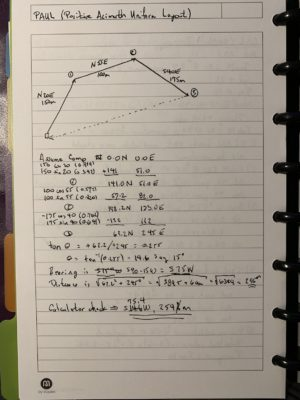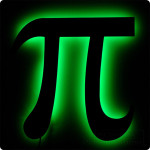# PAUL OrienteeringThis is an example of a technique for orienteering.A few days ago a video rotated onto my YouTube feed. It was a sample of field exercises taught by the Pathfinder School. One that caught my attention was the Positive Azimuth Uniform Layout method for orienteering. When I saw them working out the solution back to camp on the ground, I immediately recognized a graphical solution to the coordinate problem that surveyors used to solve all the time (before GPS surveying).

The approach is based on the conversion of bearing (or azimuth) and distance to northing and easting coordinates. When I learned to survey (with a 20-second transit, a 100-foot steel tape, a dumpy level, and a three-section level rod) this was how we did the calculations. Therefore, I wondered if I could use a slide rule to do the trigonometry, squares, and square roots required to obtain an arithmetic solution. The figure is the result of that with one error — I forgot to convert the angle (from the east-west axis) to a bearing (from the north-south axis). The error is corrected in the figure.

I also ran the solution with a hand calculator. (I used an HP-35s for the vector addition.) The calculations can be done with a pencil, piece of paper, and a slide rule. I used a Versalog 1461 (six-inch version of the Versalog slide rule). The solution is plenty good enough to return to camp.

That was fun.

# Linear Interpolation

Back in the old days, we used tabulated values to compute values of transcendental functions so that the results could be used in subsequent computations. We had tables for logarithms, trigonometric functions, and the like. There was no calculator or computer with which to enter a value and press log10 to estimate the logarithm of the number.

The method I was taught in high school (I think, it was a long time ago) is called linear interpolation. The assumption is that a straight line between entries in the table is a reasonable approximation for values in between.

That is not strictly true, because all of those functions are actually curves and deviate from a straight line between any two points on the function (according to the graph of the function). But, use of interpolation is certainly sufficient for scientific and engineering calculations.

Therefore, I learned to use linear interpolation to estimate the in-between values. The process is simple, computationally. The bounding values from the table are used to estimate a slope of the line between the two values. That slope multiplied by the fraction of the distance between the interval endpoints of the desired value of the independent variable is added to the initial interval endpoint of the dependent variable. The result is the estimate of the dependent variable on the bounding interval.

Probably 20-years ago I was working on a spreadsheet to route a runoff hydrograph through a reservoir. The algorithm is called modified Puls and is an expression of the conservation of mass principle of the hydrograph through the reservoir with the assumption that the slope on the reservoir surface is zero (it’s flat). The process involves generation of several tables of values and then using those tables to execute the algorithm. Most of the time the desired values from the algorithm fall between tabulated values. Therefore, interpolation is required.

The brute force technique would be to hand code each cell of the spreadsheet to advance the calculation. I wanted a more general (and perhaps elegant) solution so that I would not have to hand code each cell. I thought it should be possible to write a general formula using the built-in search function of the spreadsheet over the range of values in the table.

I recall working on this for a couple of days. I was a professor, so I could afford to spend the time on the problem. I constructed a solution using a function in Microsoft Excel called VLOOKUP. I am not going into the details here (I have a white paper here), but it is sufficient to say that I did the work, solved the problem, and forgot about it.

I also forgot where I put my notes on how I constructed the solution. As it turns out, I needed (or rather wanted) to use this approach to solve an engineering problem I’m working on. I couldn’t find my notes. A couple of weeks ago I hand-coded the cells just so I could get a solution. But last it became apparent that I might have to make several solutions of the problem using the same tables.

I needed to regenerate the general solution, once again. I did this a couple of days ago. Then, yesterday morning, I woke early and was unable to go back to sleep. So, I rose, made coffee, and wrote my notes so that I would have a reference should I need to use the solution again. (Hence the production of the white paper above.)

I am reminded that there are many times we work out a problem, or we learn something that challenges us in some manner. But then, with the immediate problem solved, we move on and never take time to note what the problem was, what the constraints were, what the solution was, and what we learned. We are carried away by the pressing current of other tasks, other duties, or just the ebb and flow of life.

Then, weeks, months, years, or decades later, we find ourselves facing a similar problem that, hopefully, would have a similar solution. In a frantic whirlwind of energy, we search for the solution we know we found but cannot recollect. We know we solved the problem. We remember working on it, struggling intellectually or emotionally, and the expenditure of intense energy grinding toward the solution. Then, solution found and the immediate need passed, we remember having moved on without taking the time to reflect. We curse our impatience, or failure to document what we learned, and our stupidity.

But we know, having solved the problem once before, we can find the solution (or an equivalent solution) again. So we pick up the trail, work out the solution, and solve the problem once again.

Then what? The wise will set the brake, pause, and make note of the problem, the constraints, and the derived solution. I know that I learned my lesson on this one. Will I make the same error again? Probably. But I won’t make this same error again. I have my notes. I know how to solve this problem.

What about you? What is the problem you’re faced with? Is it one you solved before? Did you pause to make notes after developing the solution? Will you make the same mistake that I did?

# Estimating Distance

A couple of weeks ago, I was watching a football game with my family. It was near sunset and the sky was illuminated by the last rays of the setting sun. Far overhead, jetliners could be seen crossing the sky, their contrails bright and warm although I knew that they existed in deadly cold.

One of them was not at cruising altitude. Older Grandson asked “How high is that plane?”

“About 25,000 feet,” SiL responded. I thought it was closer to 10K AGL.

“I don’t think so; I think it’s closer to 10,000.”

A short debate ensued and it was interesting. I respect SiL’s opinion given he works with aircraft as a professional.

Later, on the road Monday, the interchange recurred to me. As I drove along, I wondered whether there was a simple way to estimate the altitude. After thinking about it a few minutes, I came up with the following.

For small angles, the angle and the sine of the angle are approximately equal. Therefore the angle subtended by the aircraft can be used to estimate its distance from the observer. If the approximate angle from the horizontal is also known, then the elevation can be estimated.

The width of a human thumb at arm’s length is about a half-degree, or thirty minutes of angle. The pinky fingertip is about half that.

As I thought about my observations, my estimate was about three of the aircraft would be the width of my pinky finger at arm’s length… or maybe half that. That would be between two and a half and five minutes of angle, or one-twelfth to one-twenty-fourth of a degree. The sight distance to the aircraft would then be 12 to 24 times the apparent width of the vehicle.

My guess is that the apparent width of that aircraft was on the order of 120–200 feet. Therefore, for S&G’s, say it was 150 feet. At twenty times the apparent width, that would be 30,000  feet. My estimate for the angle-to-horizon was less than 45 degrees, so I’ll use that as an upper limit. The sine of 45 is about 0.7. So, the elevation is about 70 percent of the length of the hypotenuse — or sight distance.

My estimate of the altitude of the aircraft is about 20,000 feet. SiL was closer to correct than I was. Trust the professional.

# Happy Pi Day, BelatedlyI’m running a day late and a dollar (or two) short. But, I wanted to write about Pi Day before too much time gets away from me.

Every year I remember Pi Day (among a few other special days). It’s a fun reminder about the beauty and power of mathematics. One definition for pi is the ratio of the circumference of a circle to its diameter. Pi is one of the transcendental numbers, that is, it is an infinite sequence of non-repeating digits. Fun stuff…

There are a number of celebrations for Pi Day, but this year I elected to go to a geocaching meet, Pi Day with the Professor down in Gardnerville. I would meet a few geocachers, celebrate a moment of transcendence, and collect a geocaching souvenier. So the Girl and I packed out a bit early to scout some locations for landscape photographs on our way to Gardnerville.

It was a fun gathering and I was there before 9:26AM. That’s the other fun part of this particular Pi Day, yesterday, at 9:26:53AM, it was Pi Day to nine decimal places (3.141592653). This occurs only once each century, and the next one will be in 2115. I will not be there for that one.

Unfortunately, the noise and energy in the small coffee house was too much for me. I left not long after the special time, saying thanks and goodbye to my fellow lovers of the outdoors and the scavenger hunts known as geocaches. It was fun, but it was also time to get home and get after other tasks.

# LaTeX?

Apparently, WordPress has a \LaTeX interface for producing mathematics and math-like symbols. If it does, then this is something I’ve wanted in my weblog for a long time.

Alright, it works. The background is white and the foreground is black (duh), so it doesn’t quite work with my current color scheme, but it will do. At least I can now produce a decent-looking equation.